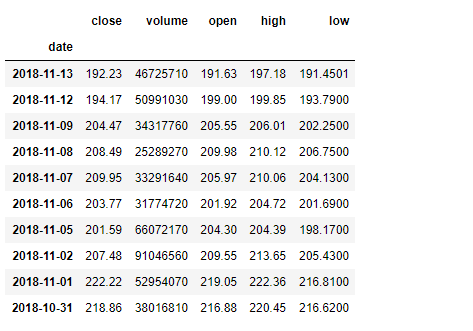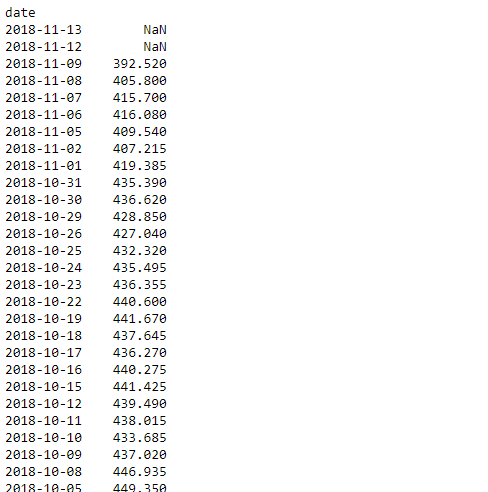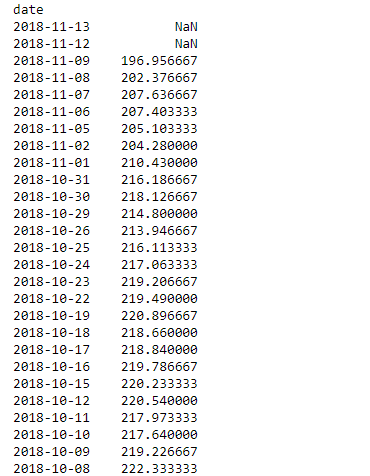Open in App
Not now

# Python | Pandas dataframe.rolling()

• Difficulty Level : Easy
• Last Updated : 21 Feb, 2022

Python is a great language for doing data analysis, primarily because of the fantastic ecosystem of data-centric python packages. Pandas is one of those packages which makes importing and analyzing data much easier.
Pandas dataframe.rolling() function provides the feature of rolling window calculations. The concept of rolling window calculation is most primarily used in signal processing and time-series data. In very simple words we take a window size of k at a time and perform some desired mathematical operation on it. A window of size k means k consecutive values at a time. In a very simple case, all the ‘k’ values are equally weighted.

Syntax : DataFrame.rolling(window, min_periods=None, freq=None, center=False, win_type=None, on=None, axis=0, closed=None)
Parameters :
window : Size of the moving window. This is the number of observations used for calculating the statistic. Each window will be a fixed size. If its an offset then this will be the time period of each window. Each window will be a variable sized based on the observations included in the time-period. This is only valid for datetimelike indexes.
min_periods : Minimum number of observations in window required to have a value (otherwise result is NA). For a window that is specified by an offset, this will default to 1.
freq : Frequency to conform the data to before computing the statistic. Specified as a frequency string or DateOffset object.
center : Set the labels at the center of the window.
win_type : Provide a window type. See the notes below.
on : For a DataFrame, column on which to calculate the rolling window, rather than the index
closed : Make the interval closed on the ‘right’, ‘left’, ‘both’ or ‘neither’ endpoints. For offset-based windows, it defaults to ‘right’. For fixed windows, defaults to ‘both’. Remaining cases not implemented for fixed windows.
axis : int or string, default 0

Note: The freq keyword is used to conform time series data to a specified frequency by resampling the data. This is done with the default parameters of resample() (i.e. using the mean).
If win_type=none, then all the values in the window are evenly weighted. There is various other types of rolling window type. To learn more about the other rolling window type refer this scipy documentation.
For a link to CSV file Used in Code, click here. This is a stock price data of Apple for a duration of 1 year from (13-11-17) to (13-11-18)
Example #1: Rolling sum with a window of size 3 on the stock closing price column

## Python3

 `# importing pandas as pd``import` `pandas as pd` `# By default the "date" column was in string format,``#  we need to convert it into date-time format``# parse_dates =["date"], converts the "date" column to date-time format` `# Resampling works with time-series data only``# so convert "date" column to index``# index_col ="date", makes "date" column``df ``=` `pd.read_csv("apple.csv", parse_dates ``=``["date"], index_col ``=``"date")` `# Printing the first 10 rows of dataframe``df[:``10``]`## Python3

 `# 3 indicates the window size``# we have selected 'triang' type window``# which returns triangular type window` `# sum() function find the sum over``# all the windows in our data frame``df.close.rolling(``3``, win_type ``=``'triang'``).``sum``()`

Output :Example #2: Rolling window mean over a window size of 3. we use default window type which is none. So all the values will be evenly weighted.

## Python3

 `# importing pandas as pd``import` `pandas as pd` `df ``=` `pd.read_csv("apple.csv", parse_dates ``=``["date"], index_col ``=``"date")` `# close is the column on which``# we are performing the operation``# mean() function finds the mean over each window``df.close.rolling(``3``).mean()`

Output :My Personal Notes arrow_drop_up